## Exercise 1

Find the point in the function y = |x + 2| where it has no derivative. Justify the result by representing it graphically.

## Exercise 2

Find the point in the function y = |x ² − 5x + 6| where it has no derivative. Justify the result by representing it graphically.

## Exercise 3

Study the continuity and differentiability of the function defined by:## Exercise 4

Given the function:For what values of a is the function differentiable?

## Exercise 5

Determine the values of a and b where the following function is continuous and differentiable: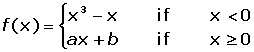## Exercise 6

Determine the values of a and b for which the function is differentiable at all points:## Exercise 7

Find the points where y = 250 − |x² −1| has no derivative.

## Exercise 8

Determine for which values of a and b the function is continuous and differentiable:## Solution of exercise 1

Find the point in the function y = |x + 2| where it has no derivative. Justify the result by representing it graphically.The function is continuous.f'(−2) = −1f'(−2)+ = 1

It has no derivative at P(−2,0).## Solution of exercise 2

Find the point in the function y = |x ² − 5x + 6| where it has no derivative. Justify the result by representing it graphically.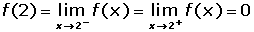The function is continuous.f'(2)- = −1f'(2)+ = 1

f'(3)- = −1f'(3)+ = 1

The function is not differentiable at: x = 2 and x = 3 or at points P1(2,0) and P2(3,0).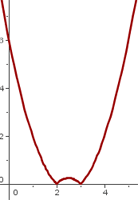## Solution of exercise 3

Study the continuity and differentiability of the function defined by:The function is not continuous at x = 0 because it has no image. Therefore it is not differentiable.The function is continuous.The function is not differentiable at any point.

## Solution of exercise 4

Given the function: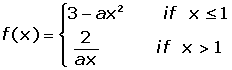For what values of a is the function differentiable?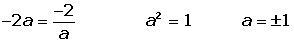Differentiable at a = 1

For x = −1, it is not continuous.

## Solution of exercise 5

Determine the values of a and b where the following function is continuous and differentiable: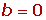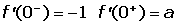## Solution of exercise 6

Determine the values of a and b for which the function is differentiable at all points:A differentiable function has to be continuous. In this case the function is not continuous for x = 0, that is to say, there are no values for a and b which make the function continuous.

Therefore, there are no values of a and b for which the function is differentiable.

## Solution of exercise 7

Find the points where y = 250 − |x² −1| has no derivative.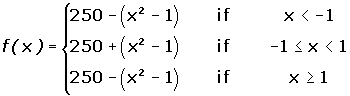The function is continuous.Is not differentiable at x = −1 and x = 1.

## Solution of exercise 8

Determine for which values of a and b the function is continuous and differentiable:For a = 1 and b = 4, the function is continuous.It is not differentiable at x = 0.It is differentiable at x = 2.

Did you like the article?(1 votes, average: 5.00 out of 5)Loading...

Emma

I am passionate about travelling and currently live and work in Paris. I like to spend my time reading, gardening, running, learning languages and exploring new places.

Did you like
this resource?

Bravo!